# Electric potential energy of charges in external electric field. [definition & formula].

• 1
•
•
•
•
•
•
•
1
Share

In this article we will understand and derive the expression for potential energy of charges in external electric field. so keep reading…

# ELECTRIC POTENTIAL ENERGY

Before going further let’s revise the concept of electric potential energy. If we see the term electric potential energy, then we see that this term is self explanatory.

We can define this term as –  It is the energy which is stored in the electrical bodies when it is moved from one point to another point in any external electric field. In other words we can say that – It is the energy acquired  by the charged bodies by virtue of change in its position in any external electric field.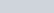Where V is the electric potential at that point.

There are lots of ways by which we can define electric potential energy. A famous definition is – It is the amount of energy acquired by the charge particle in bringing a positive charge from infinity to a specific point in any electric field without any acceleration.

## ELECTRIC POTENTIAL ENERGY OF A SINGLE CHARGE IN EXTERNAL ELECTRIC FIELD

Let’s consider a charge particle q placed in external electric field of magnitude E. Here we consider this charge particle very small. Then the potential energy of this charge particle q in this external electric field is equal to the work done in bringing this charge particle from infinity to a specific point in electric field.

Keep in mind that value of electric field and electric potential can vary from point to point because these are dependent on the position vector r.

The value of electric potential energy of charge particle q in external electric field at position vector r from the origin is given as-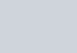Where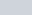is electric potential at position r from the origin.

## ELECTRIC POTENTIAL ENERGY OF A SYSTEM OF TWO POINT CHARGE IN EXTERNAL ELECTRIC FIELD

Let’s consider a system of two charges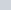and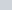, which is placed at position vector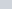and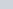from the origin in electric field of magnitude E.

Now what is the potential energy acquired by this system of two charge particles?  Let the work done in bringing the chargefrom infinity to position vectoris given as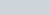and work done in bringing the chargefrom infinity to the position vectoris given as.

But here we have to note one thing that when are we bringing chargefrom infinity to the position vectorthen we have to do work against the two opposing factors, first is electric field and second is electric field due to charge. So we have to do little more work in bringing chargefrom infinity to the position. So the potential energy acquired by the chargedue to chargeis given as-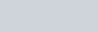Where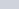is the position vector between chargeand. But the total work done in bringing chargefrom infinity to position vectoris given as-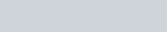Now the total work done in bringing both the electric charges from infinity to the present configuration is given as-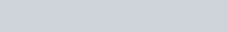Watch this video for more reference: